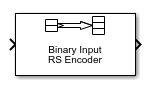# Binary-Input RS Encoder

Create Reed-Solomon code from binary vector data

• Library:
• Communications Toolbox / Error Detection and Correction / Block

•## Description

The Binary-Input RS Encoder block creates a Reed-Solomon code.

The symbols for the code are binary sequences of length M, corresponding to elements of the Galois field GF(2M). The first bit in each symbol is the most significant bit.

Suppose M = 3, N = 23-1 = 7, and K = 2. Then a message is a vector of length 2 whose entries are integers between 0 and 7. A corresponding codeword is a vector of length 7 whose entries are integers between 0 and 7. The following figure illustrates possible input and output signals to this block when codeword length N=7 and message word length K=2. Since N=2M–1, when N=7, the symbol length, M=3.Each input message word is a binary vector of length 6, that represents 2 three-bit integers. Each corresponding output codeword is a binary vector of length 21 that represents 7 three-bit integers. For more information, see Input and Output Signal Length in RS Blocks.

## Ports

### Input

expand all

Message in bits, specified as one of the following:

• When there is no message shortening, a (NC×K×M)-by-1 binary column vector.

• When there is message shortening, a (NC×S×M)-by-1 binary column vector.

NC is the number of message words, K is the Message length K (symbols), M is the number of bits per symbol, and S is the Shortened message length S (symbols).

Note

The number of decoded message words equals the number of codewords.

For more information, see Input and Output Signal Length in RS Blocks.

Data Types: `single` | `double` | `int8` | `int16` | `int32` | `uint8` | `uint16` | `uint32` | `Boolean` | `ufix(1)`

### Output

expand all

Reed-Solomon codeword in bits, returned as an (NC×(NK + SPM)-by-1 binary column vector. NC is the number of codewords, N is the Codeword length N (symbols), K is the Message length K (symbols), S is the Shortened message length S (symbols), P is the number of punctures per codeword, and M is the number of bits per symbol.

For more information, see Input and Output Signal Length in RS Blocks.

Data Types: `single` | `double` | `int8` | `int16` | `int32` | `uint8` | `uint16` | `uint32` | `Boolean` | `ufix(1)`

## Parameters

expand all

Codeword length in symbols, specified as an integer.

For more information, see Restrictions on the M and the Codeword Length N and Input and Output Signal Length in RS Blocks.

Message word length in symbols, specified as an integer in the range [1, N–2], where N is the codeword length.

Shortened message word length in symbols, specified as an integer, such that SK. When Shortened message length S (symbols) < Message length K (symbols), the Reed-Solomon code is shortened.

You still specify N and K values for the full-length (N, K) code but the decoding is shortened to an (NK+S, S) code.

#### Dependencies

To enable this parameter, select .

Generator polynomial with values from 0 to 2M–1, in order of descending power, specified as one of the following:

• A polynomial character vector. For more information, see Representation of Polynomials in Communications Toolbox.

• An integer row vector that represents the coefficients of the generator polynomial in order of descending power.

• An integer Galois row vector that represents the coefficients of the generator polynomial in order of descending power.

Each coefficient is an element of the Galois field defined by the primitive polynomial. For more information, see Specify the Generator Polynomial.

Example: `[1 3 1 2 3]`, which is equivalent to `rsgenpoly(7,3)`

#### Dependencies

To enable this parameter, select .

Primitive polynomial in order of descending power. This polynomial is of order M and defines the finite Galois field GF(2M) corresponding to the integers that form message words and codewords. Specify the primitive polynomial as one of the following:

For more information, see Restrictions on the M and the Codeword Length N.

Example: `'X^3 + X + 1'`, which is the primitive polynomial used for a (7,3) code, ```ppoly = primpoly(3,'nodisplay'); int2bit(ppoly,ceil(log2(max(ppoly))))'```

#### Dependencies

To enable this parameter, select .

Puncture vector, specified as an (NK)-by-1 binary column vector. Element indices with `1`s represent data symbol indices that pass through the block unaltered. Element indices with `0`s represent data symbol indices that get punctured, or removed, from the data stream. For more information, see Puncturing and Erasures.

#### Dependencies

To enable this parameter, select .

Output type of the block, specified as ```Same as input```, `boolean`, or `double`.

## Block Characteristics

 Data Types `Boolean` | `double` | `fixed point[a]` | `integer` | `single` Multidimensional Signals `no` Variable-Size Signals `no` [a] ufix(1) only.

expand all

## Algorithms

This object implements the algorithm, inputs, and outputs described in Algorithms for BCH and RS Errors-only Decoding.

## Extended Capabilities

### Functions

Introduced before R2006a